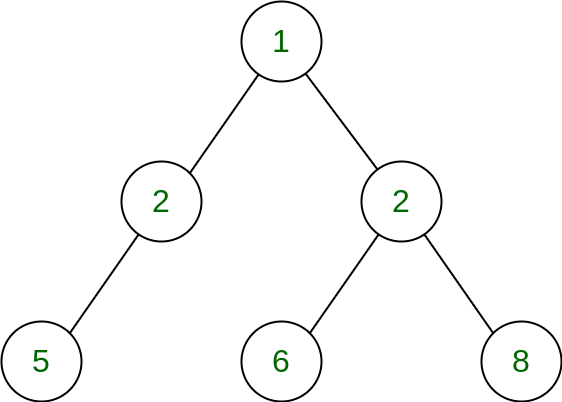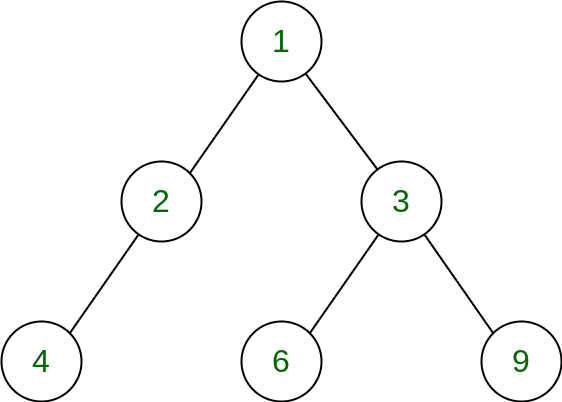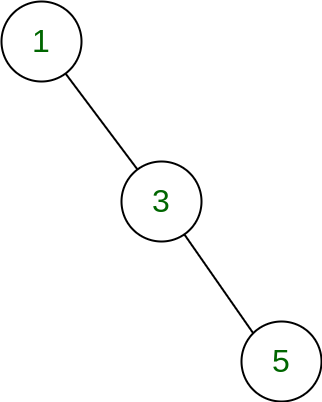# Minimize changes to convert into Tree with root 1, even left children and odd right children

• Last Updated : 16 Jun, 2022

Given, a binary tree, the task is to convert this tree using minimum number of increment-decrement operations into a tree which satisfies the following conditions:

• The root node is always 1.
• Every left child of a node is even.
• Every right child of a node is odd.

Return and print the minimum number of increment-decrement operations required at the end.

Examples:

Input:Output: 3
Explanation:
Since root is already 1, no change is needed at root
Left child of root node is 2, so no change is needed here.
Right child of root node is 2, so change it to 3, making a change of 1.
Left child of node 2 is 5, so change it to 4, making a change of 1.
Left child of node 3 is 6, so no change is needed here.
Right child of node 3 is 8, so change it to 9, making a change of 1.
Hence total changes needed is 3.Input:Output: 0
Explanation: The given tree already satisfies the given conditions.

Approach: The idea is to change every node which does not satisfies the condition based on below observation:

• If the root node is not 1, we can keep decrementing it by 1 till it becomes 1. So root-1 operations needed here.
• If the current node is a left child, and it is odd, we can simply make it even by incrementing or decrementing by 1. So 1 operation is needed here.
• If the current node is a right child, and it is even, we can simply make it odd by incrementing or decrementing by 1. So 1 operation is needed here.

Therefore, simply traverse the given Tree and:

• Add root-1 operations to the answer if the root is not 1.
• Add the count of left child which are odd, to the answer
• Also add the count of right child which are even, to the answer.

Based on above idea, we can do a DFS traversal as per below steps:

• Traverse left and right child recursively.
• Check if visited node’s left value is not null and node’s left child value is odd
• Check if visited node’s right value is not null and node’s right child value is even
• Again Recursively call for left and right node till whole tree is traversed.
• Also check if root is not equal to 1.
• Return the answer at the end.

Below is the implementation of the above approach:

## C++

 `// C++ code for the above approach` `#include ``using` `namespace` `std;` `// To store the final changes needed``int` `count_of_changes;` `struct` `Node {``    ``int` `value;``    ``struct` `Node *left, *right;``};` `// Utility function to create new``// tree node``Node* newNode(``int` `data)``{``    ``Node* temp = ``new` `Node;``    ``temp->value = data;``    ``temp->left = temp->right = NULL;``    ``return` `temp;``}` `// DFS function to convert``// binary tree to proper tree``void` `dfs(Node* root)``{` `    ``// Check if given root is NULL``    ``// base case``    ``if` `(root == NULL)``        ``return``;` `    ``// Check if visited node's``    ``// left value is not null and``    ``// node's left child value is odd``    ``// decrement its value by 1``    ``if` `(root->left``        ``&& root->left->value % 2 == 1) {``        ``root->left->value -= 1;``        ``count_of_changes++;``    ``}` `    ``// Check if visited node's``    ``// value is not null and node's``    ``// right child value is even,``    ``// increment its value by 1``    ``if` `(root->right``        ``&& root->right->value % 2 == 0) {``        ``root->right->value += 1;``        ``count_of_changes++;``    ``}` `    ``// Recursive call for left node``    ``dfs(root->left);` `    ``// Recursive call for right node``    ``dfs(root->right);``}` `// Function to find``// the min changes needed``int` `minCount(Node* root)``{` `    ``// Initial value for``    ``// final changes needed``    ``count_of_changes = 0;` `    ``// Base case to check``    ``// if root is NULL``    ``if` `(root == NULL)``        ``return` `count_of_changes;` `    ``if` `(root->value != 1) {` `        ``// Add root_value - 1 to the ans``        ``count_of_changes += root->value - 1;` `        ``// Set root->Value to 1``        ``root->value = 1;``    ``}` `    ``// DFS Function call``    ``dfs(root);` `    ``// Return the final count``    ``return` `count_of_changes;``}` `// Driver Code``int` `main()``{` `    ``// Taking input``    ``Node* root = newNode(1);``    ``root->left = newNode(2);``    ``root->right = newNode(2);``    ``root->left->left = newNode(5);``    ``root->right->left = newNode(6);``    ``root->right->right = newNode(8);` `    ``// Function call``    ``cout << minCount(root) << endl;` `    ``return` `0;``}`

## Java

 `// JAVA code for the above approach``import` `java.util.*;``class` `GFG``{` `  ``// To store the final changes needed``  ``static` `int` `count_of_changes;` `  ``public` `static` `class` `Node {``    ``int` `value;``    ``Node left, right;``  ``}` `  ``// Utility function to create new``  ``// tree node` `  ``public` `static` `Node newNode(``int` `data)``  ``{``    ``Node temp = ``new` `Node();``    ``temp.value = data;``    ``temp.left = temp.right = ``null``;``    ``return` `temp;``  ``}``  ``// DFS function to convert``  ``// binary tree to proper tree``  ``public` `static` `void` `dfs(Node root)``  ``{` `    ``// Check if given root is null``    ``// base case``    ``if` `(root == ``null``)``      ``return``;` `    ``// Check if visited node's``    ``// left value is not null and``    ``// node's left child value is odd``    ``// decrement its value by 1``    ``if` `(root.left != ``null` `&& root.left.value % ``2` `== ``1``) {``      ``root.left.value -= ``1``;``      ``count_of_changes++;``    ``}` `    ``// Check if visited node's``    ``// value is not null and node's``    ``// right child value is even,``    ``// increment its value by 1``    ``if` `(root.right != ``null` `&& root.right.value % ``2` `== ``0``) {``      ``root.right.value += ``1``;``      ``count_of_changes++;``    ``}` `    ``// Recursive call for left node``    ``dfs(root.left);` `    ``// Recursive call for right node``    ``dfs(root.right);``  ``}` `  ``// Function to find``  ``// the min changes needed``  ``public` `static` `int` `minCount(Node root)``  ``{` `    ``// Initial value for``    ``// final changes needed``    ``count_of_changes = ``0``;` `    ``// Base case to check``    ``// if root is null``    ``if` `(root == ``null``)``      ``return` `count_of_changes;` `    ``if` `(root.value != ``1``) {` `      ``// Add root_value - 1 to the ans``      ``count_of_changes += root.value - ``1``;` `      ``// Set root->Value to 1``      ``root.value = ``1``;``    ``}` `    ``// DFS Function call``    ``dfs(root);` `    ``// Return the final count``    ``return` `count_of_changes;``  ``} `  `  ``// Driver code``  ``public` `static` `void` `main(String[] args)``  ``{``    ``// Taking input``    ``Node root = newNode(``1``);``    ``root.left = newNode(``2``);``    ``root.right = newNode(``2``);``    ``root.left.left = newNode(``5``);``    ``root.right.left = newNode(``6``);``    ``root.right.right = newNode(``8``);` `    ``// Function call``    ``System.out.println(minCount(root));` `  ``}``}` `// This code is contributed by jana_sayantan.`

## Python3

 `# Python code for the above approach` `# To store the final changes needed``count_of_changes ``=` `0` `class` `Node:``    ``def` `__init__(``self``,data ``=` `0``,left ``=` `None``,right ``=` `None``):``        ``self``.data ``=` `data``        ``self``.left ``=` `left``        ``self``.right ``=` `right` `# Utility function to create new``# tree node``def` `newNode(data):` `    ``temp ``=` `Node()``    ``temp.value ``=` `data``    ``temp.left ``=` `temp.right ``=` `None``    ``return` `temp`  `# DFS function to convert``# binary tree to proper tree``def` `dfs(root):` `    ``global` `count_of_changes` `    ``# Check if given root is None``    ``# base case``    ``if` `(root ``=``=` `None``):``        ``return` `    ``# Check if visited node's``    ``# left value is not None and``    ``# node's left child value is odd``    ``# decrement its value by 1``    ``if` `(root.left ``and` `root.left.value ``%` `2` `=``=` `1``) :``        ``root.left.value ``-``=` `1``        ``count_of_changes ``+``=` `1` `    ``# Check if visited node's``    ``# value is not None and node's``    ``# right child value is even,``    ``# increment its value by 1``    ``if` `(root.right``        ``and` `root.right.value ``%` `2` `=``=` `0``):``        ``root.right.value ``+``=` `1``        ``count_of_changes ``+``=` `1` `    ``# Recursive call for left node``    ``dfs(root.left)` `    ``# Recursive call for right node``    ``dfs(root.right)` `# Function to find``# the min changes needed``def` `minCount(root):` `    ``# Initial value for``    ``# final changes needed``    ``global` `count_of_changes``    ``count_of_changes ``=` `0` `    ``# Base case to check``    ``# if root is None``    ``if` `(root ``=``=` `None``):``        ``return` `count_of_changes` `    ``if` `(root.value !``=` `1``):` `        ``# Add root_value - 1 to the ans``        ``count_of_changes ``+``=` `root.value ``-` `1` `        ``# Set root.Value to 1``        ``root.value ``=` `1` `    ``# DFS Function call``    ``dfs(root)` `    ``# Return the final count``    ``return` `count_of_changes` `# Driver Code` `# Taking input``root ``=` `newNode(``1``)``root.left ``=` `newNode(``2``)``root.right ``=` `newNode(``2``)``root.left.left ``=` `newNode(``5``)``root.right.left ``=` `newNode(``6``)``root.right.right ``=` `newNode(``8``)` `# Function call``print``(minCount(root))` `# This code is contributed by shinjanpatra`

## C#

 `// C# program to implement above approach``using` `System;``using` `System.Collections;``using` `System.Collections.Generic;` `class` `GFG``{` `  ``// To store the final changes needed``  ``static` `int` `count_of_changes;` `  ``public` `class` `Node {``    ``public` `int` `value;``    ``public` `Node left, right;``  ``}` `  ``// Utility function to create new``  ``// tree node` `  ``public` `static` `Node newNode(``int` `data)``  ``{``    ``Node temp = ``new` `Node();``    ``temp.value = data;``    ``temp.left = temp.right = ``null``;``    ``return` `temp;``  ``}` `  ``// DFS function to convert``  ``// binary tree to proper tree``  ``public` `static` `void` `dfs(Node root)``  ``{` `    ``// Check if given root is null``    ``// base case``    ``if` `(root == ``null``)``      ``return``;` `    ``// Check if visited node's``    ``// left value is not null and``    ``// node's left child value is odd``    ``// decrement its value by 1``    ``if` `(root.left != ``null` `&& root.left.value % 2 == 1) {``      ``root.left.value-=1;``      ``count_of_changes++;``    ``}` `    ``// Check if visited node's``    ``// value is not null and node's``    ``// right child value is even,``    ``// increment its value by 1``    ``if` `(root.right != ``null` `&& root.right.value % 2 == 0) {``      ``root.right.value += 1;``      ``count_of_changes++;``    ``}` `    ``// Recursive call for left node``    ``dfs(root.left);` `    ``// Recursive call for right node``    ``dfs(root.right);``  ``}` `  ``// Function to find``  ``// the min changes needed``  ``public` `static` `int` `minCount(Node root)``  ``{` `    ``// Initial value for``    ``// final changes needed``    ``count_of_changes = 0;` `    ``// Base case to check``    ``// if root is null``    ``if` `(root == ``null``)``      ``return` `count_of_changes;` `    ``if` `(root.value != 1) {` `      ``// Add root_value - 1 to the ans``      ``count_of_changes += root.value - 1;` `      ``// Set root->Value to 1``      ``root.value = 1;``    ``}` `    ``// DFS Function call``    ``dfs(root);` `    ``// Return the final count``    ``return` `count_of_changes;``  ``}`  `  ``public` `static` `void` `Main(``string``[] args){` `    ``// Taking input``    ``Node root = newNode(1);``    ``root.left = newNode(2);``    ``root.right = newNode(2);``    ``root.left.left = newNode(5);``    ``root.right.left = newNode(6);``    ``root.right.right = newNode(8);` `    ``// Function call``    ``Console.WriteLine(minCount(root));` `  ``}``}` `// This code is contributed by entertain2022.`

## Javascript

 ``

Output

`3`

Time Complexity: O(V), where V is the count of vertices in given Tree
Auxiliary Space: O(H), which is the size of the stack for function calls, where H is the height of the tree.

My Personal Notes arrow_drop_up